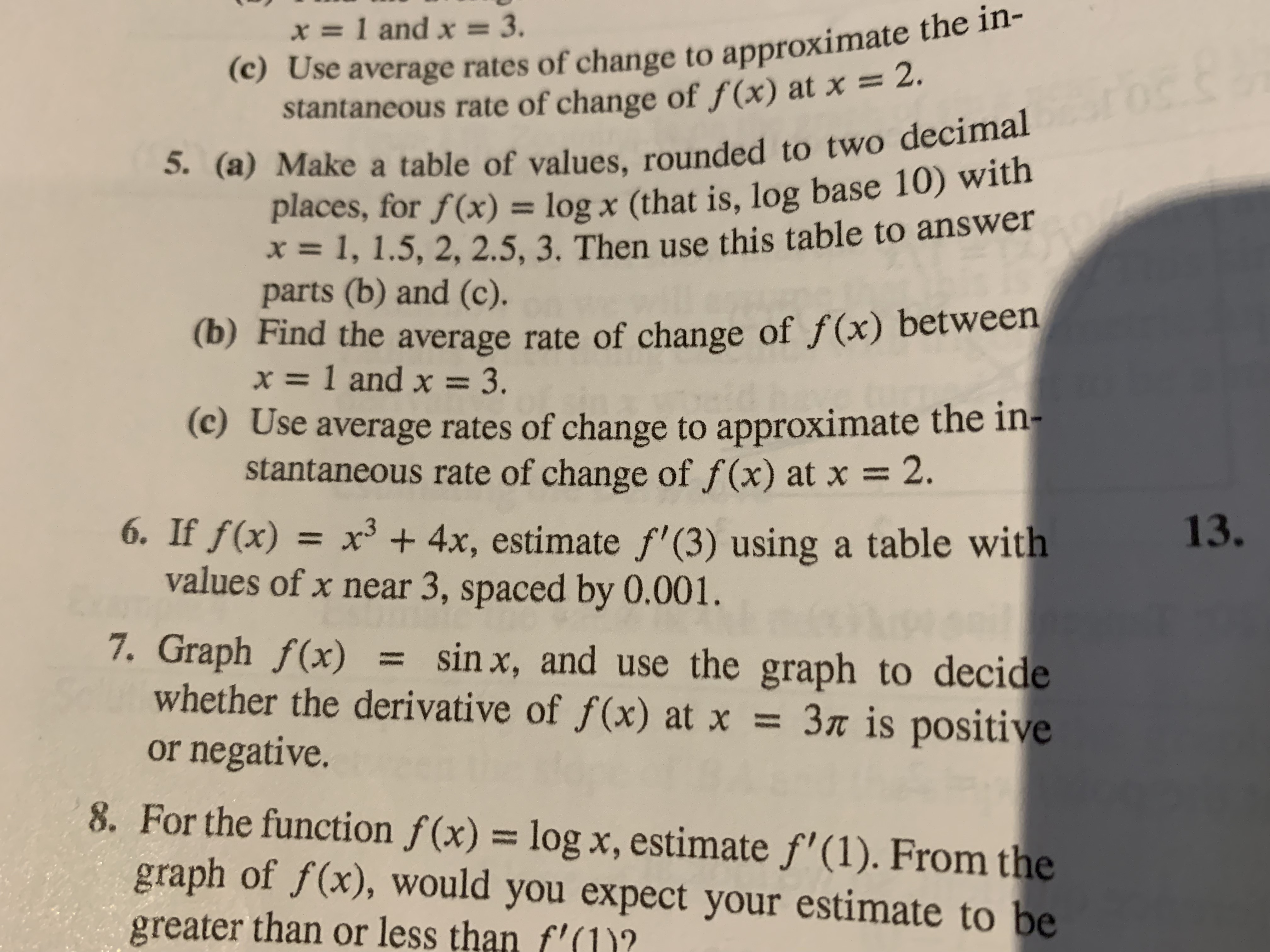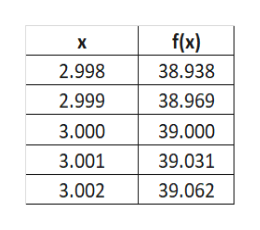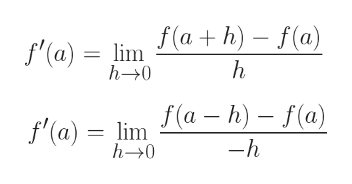(c) Use average rates of change to approximate the in-stantaneous rate of change of f(x) at x = 2.5. (a) Make a table of values, rounded to two decimalplaces, for f(x) = log x (that is, log base 10) with=1, 1.5, 2, 2.5, 3. Then use this table to answerparts (b) and (c).(b) Find the average rate of change of f(x) between=1 and x 3.(c) Use average rates of change to approximate the in-stantaneous rate of change of f (x) at xx=1 and x = 3.2.6. If f(x) = x3 4x, estimate f' (3) using a table withvalues of x near 3, spaced by 0.001.7. Graph f(x)whether the derivative of f(x) at x = 3« is positiveor negative.13.sin x, and use the graph to decide8. For the function f(x) = log x, estimate f'(1). From thegraph of f(x), would you expect your estimate to begreater than or less than f'(1)?

Questionhelp_outlineImage Transcriptionclose(c) Use average rates of change to approximate the in- stantaneous rate of change of f(x) at x = 2. 5. (a) Make a table of values, rounded to two decimal places, for f(x) = log x (that is, log base 10) with =1, 1.5, 2, 2.5, 3. Then use this table to answer parts (b) and (c). (b) Find the average rate of change of f(x) between =1 and x 3. (c) Use average rates of change to approximate the in- stantaneous rate of change of f (x) at x x=1 and x = 3. 2. 6. If f(x) = x3 4x, estimate f' (3) using a table with values of x near 3, spaced by 0.001. 7. Graph f(x) whether the derivative of f(x) at x = 3« is positive or negative. 13. sin x, and use the graph to decide 8. For the function f(x) = log x, estimate f'(1). From the graph of f(x), would you expect your estimate to be greater than or less than f'(1)? fullscreen
Step 1

f(x) = x3 + 4x

Hence, we can have the table for values of f(x) in the vicinity of x = 3 as shown on the white board.help_outlineImage Transcriptionclosef(x) X 2.998 38.938 2.999 38.969 3.000 39.000 3.001 39.031 3.002 39.062 fullscreen
Step 2

Let\'s now define a = 3. Let\'s recall the formula for right hand derivative and left han...help_outlineImage Transcriptionclosef'(a) lima h) - f(a) h h-0 f'(a) = lima-h)- f(a) -h h-0 fullscreen

Want to see the full answer?

See Solution

Want to see this answer and more?

Our solutions are written by experts, many with advanced degrees, and available 24/7

See Solution
Tagged in

Calculus